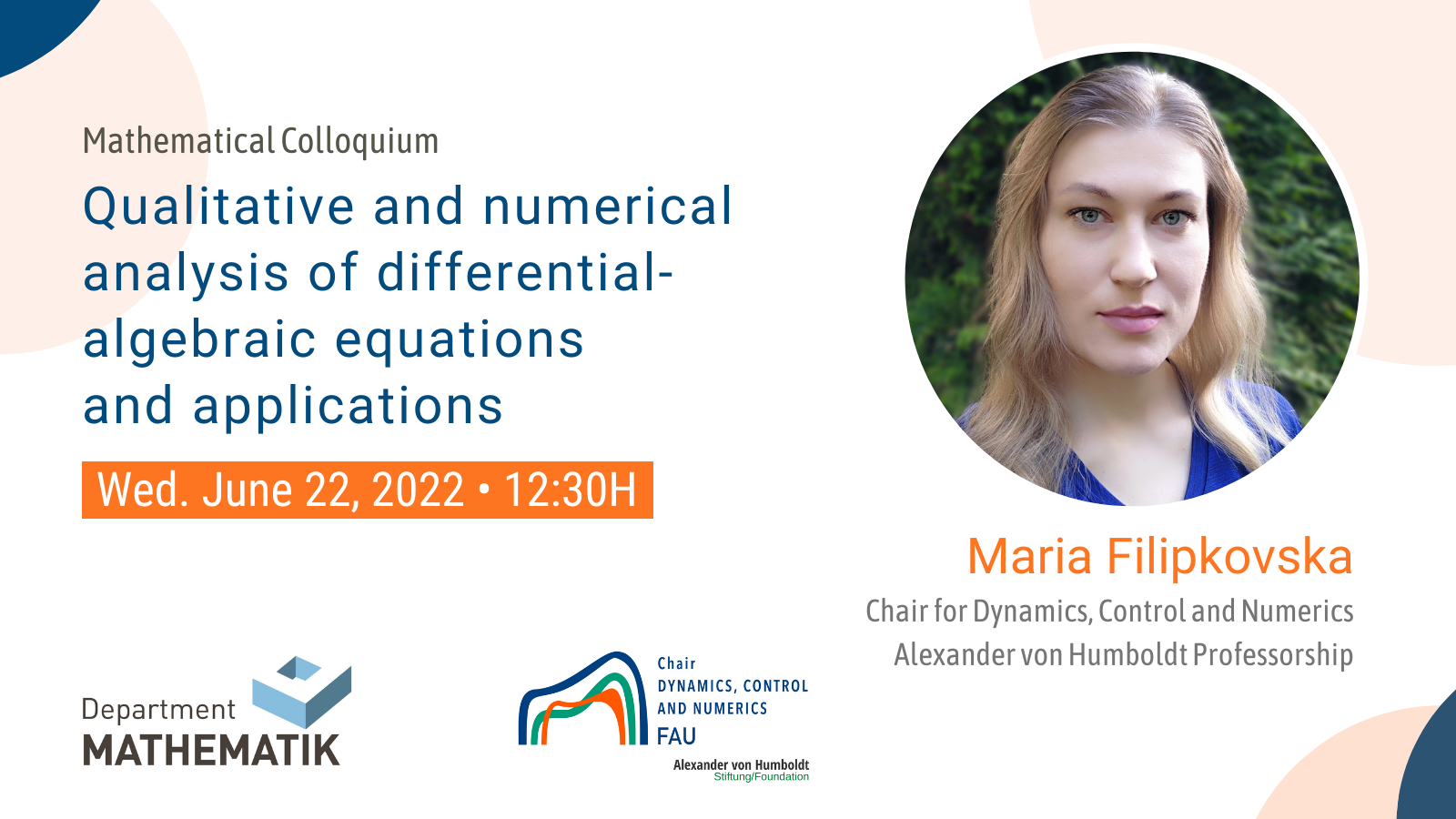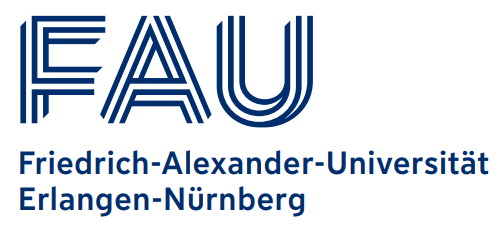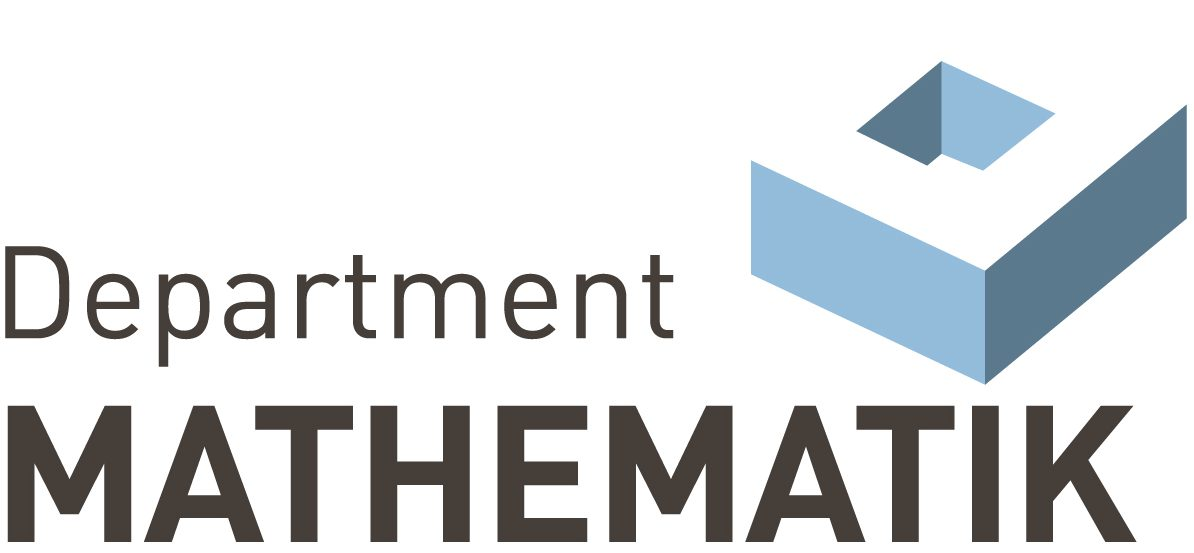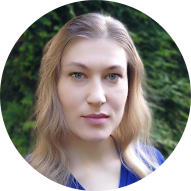# Mathematical Colloquium: Qualitative and numerical analysis of differential-algebraic equations, and applications

On June 22nd. our postdoctoral researcher Maria Filipkovska will talk on “Qualitative and numerical analysis of differential-algebraic equations, and applications” at the Mathematical Colloquium organized by the Department of Mathematik at Friedrich-Alexander-Universität Erlangen-Nürnberg.

Abstract. Differential-algebraic equations (DAEs) or degenerate DEs appear as mathematical models in control theory, where they are called descriptor systems, radioelectronics, cybernetics, mechanics, robotics technology, economics, ecology and chemical kinetics. DAEs are particularly used in modeling various networks such as gas networks. A feature of a DAE is that the graphs of its solutions lie in the manifold which is determined by the algebraic part of the equation. For time-varying semilinear DAEs, theorems on the global solvability, the Lagrange and Lyapunov stability and the dissipativity are presented. We state numerical methods for solving semilinear DAEs and discuss applications of the obtained results. Certain mathematical models for electrical circuits with nonlinear and time-varying elements are considered in detail.

#### WHERE?

Online:
Meeting ID: 695 6013 9382 | PIN: 982205

On-site:
Room H13. Department of Mathematik.
Friedrich-Alexander-Universität Erlangen-Nürnberg (FAU)
Cauestrasse 11, 91058 Erlangen

### Date

Wed. Jun 22, 2022
Expired!

### Time

12:30 - 13:30### Location

Category### Speaker

•Maria Filipkovska